Next: Practical implementation Up: The generic analyzer Previous: The generic analyzer   Contents

Principle

The purpose of the generic analyzer is to try to refine a box and to propose smaller sub-intervals which may contain real roots of the system of equations (which may be algebraic or not). Two types of tools are used for that purpose:
• as soon as one of the equation in the system is algebraic in at least one of the unknowns then the tools described for determining ranges on the roots of an univariate polynomial with interval coefficients are used recursively
• otherwise Kantorovitch theorem is used (if you have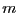equations andunknowns with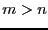, then this theorem is applied only for the firstequations)
More precisely consider the system in two unknowns: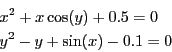In a first step the first equation is considered: it will be considered as a polynomial in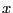with the interval coefficients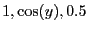. Bounds on the real roots of this polynomial will be computed and the range onwill be updated if necessary. Then the second equation will be considered as a polynomial inwith interval coefficients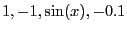Bounds on the real roots of this polynomial will be computed and the range onwill be updated if necessary. After these two steps if a range has been improved we will reiterate the process until no further improvement is obtained.

We will then try to improve the obtained ranges by using Kantorovitch theorem. We consider the middle point of the ranges and test if Kantorovitch is able to determine a ball centered at this point for which Newton method will converge toward the unique solution in the ball. If the answer is positive we will use Newton with as starting point the center of the ball: the result, a point, is stored as one of the result ranges. The intersection of the ball with the initial range will then be computed and if non empty will be stored for further processing.

We may indeed perform an in-depth analysis. The previous process is applied on the initial range and will lead to a set of ranges (possibly to the same ranges than the initial one); we may then bisect the obtained ranges and reiterate the process on the resulting ranges, discarding those for which the interval evaluation for at least one of the equations does not include 0. We define a depth level for the algorithm as the number of bisection steps that will be used in the process (a depth 0 means that no bisection will be done).Next: Practical implementation Up: The generic analyzer Previous: The generic analyzer   Contents
Jean-Pierre Merlet 2012-12-20